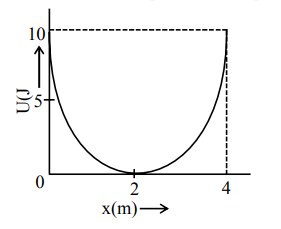# A mass of 5 kg is connected to a spring.Question:

A mass of 5 kg is connected to a spring. The potential energy curve of the simple harmonic motion executed by the system is shown in the figure. A simple pendulum of length 4 m has the same period of oscillation as the spring system. What is the value of acceleration due to gravity on the planet where these experiments are performed?1. $10 \mathrm{~m} / \mathrm{s}^{2}$

2. $5 \mathrm{~m} / \mathrm{s}^{2}$

3. $4 \mathrm{~m} / \mathrm{s}^{2}$

4. $9.8 \mathrm{~m} / \mathrm{s}^{2}$

Correct Option: , 3

Solution:

From potential energy curve

$U_{\max }=\frac{1}{2} \mathrm{kA}^{2} \Rightarrow 10=\frac{1}{2} \mathrm{k}(2)^{2}$

$\Rightarrow \mathrm{k}=5$

Now $\mathrm{T}_{\text {spring }}=\mathrm{T}_{\text {pendulum }}$

$2 \pi \sqrt{\frac{5}{5}}=2 \pi \sqrt{\frac{4}{g}}$

$\Rightarrow 1=\sqrt{\frac{4}{g}} \Rightarrow g=4$ on planet

Option (3)